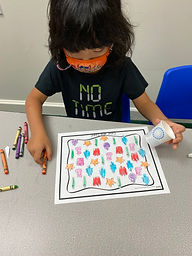Ms. Brianne

Target 1​

Lesson Type:

Continuation

Number Operation

:

Integer Composition

Understand that the two digits in a double-digit number represent the number of tens and ones.

1:

Represent the composition or decomposition of two-digit numbers with an equation (13 = 10+3 or 1 ten and 3 ones).

1st

Vocabulary:

Place Value, Tens, Ones

Activities:

• Students represented a given number with base-ten blocks and then wrote the matching equation.
• Students wrote the number of tens and ones shown in a picture to determine the two-digit number it represented.
• Students were given a card with a quantity of base-ten blocks and found the matching numeral.
• Students created a number ornament by writing their numeral on the top, and pasting tens and ones on the ornament to show how many tens and ones compose the number.Home Exploration

Guiding Questions:Absent Students:

Isabella, Zia, Haven, Elise

Target 2

:

1:

Looking at a picture where information is chaotic or spread out, count to gather data to answer questions.

2:

Understand that data can be read more quickly in graph form.

1st

Vocabulary:

Data, Bar Graph, Greater, Less

Activities:

• Students were given a set of unorganized data. They colored and counted to gather data about the number of each object shown. Then they represented the data in a bar graph.
• Students used their bar graph to help them answer questions about the data.Home Exploration

Guiding Questions:Target 3

:

Vocabulary:

Activities:Home Exploration